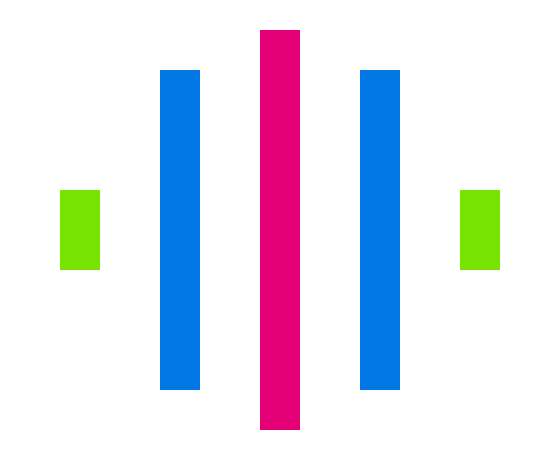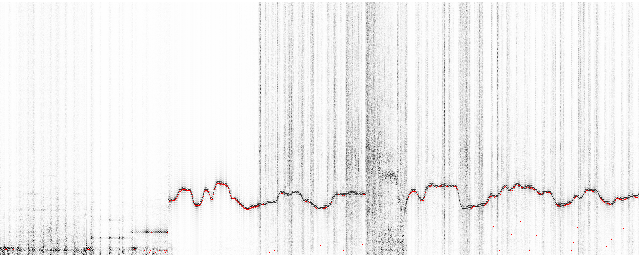# ~ Tarsos Spectrogram

Today I created a spectrogram application using Tarsos. The application listens to an audio input, computes an FFT and at the same time calculates pitch. The expected pitch is overlaid on the spectrogram. All this happens real-time and is implemented using JAVA.This is the most recent version of the spectrogram implementation in java.

``````
1
2
3
4
5
6
7
8
9
10

float pitch = Yin.processBuffer(buffer, (float) sampleRate);
fft.transform(buffer);
double maxAmplitude = 0;
for (int j = 0; j < buffer.length / 2; j++) {
double amplitude = buffer[j] * buffer[j] + buffer[j +
buffer.length/2] * buffer[j+ buffer.length/2];
amplitude = Math.pow(amplitude, 0.5);
colorIndexes[j] = amplitude;
maxAmplitude = Math.max(amplitude, maxAmplitude);
}

``````

If you want to test it yourself download the spectrogram jar package and execute:

``````
1

java -jar spectrogram.jar

``````